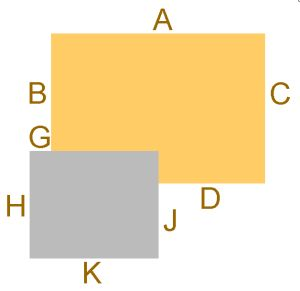01603 721590
Information
Search
UK
Set Delivery
Postcode
CO2
Empty
Setting the first half of your postcode shows you the full choice in your area.

# Base dimensions

## 5.0m deep cabins (16'5")We recommend that your base should be no smaller than the floor size of the new log cabin and no more than a few inches larger.

Cabin size - 16'5" x 13'1" (5.0m x 4.0m)
Dimension A = 4000mm
Dimension B = 4000mm
Dimension C = 5000mm
Dimension D = 2000mm
Dimension G = 1000mm
Dimension H = 3000mm
Dimension J = 2000mm
Dimension K = 3000mm

Overall width from side to side (A) = 4000mm (excluding verandah)
Overall depth from front to back (C) = 5000mm (excluding verandah)

Cabin size - 16'5" x 14'9" (5.0m x 4.5m)
Dimension A = 4500mm
Dimension B = 4000mm
Dimension C = 5000mm
Dimension D = 2500mm
Dimension G = 1000mm
Dimension H = 3000mm
Dimension J = 2000mm
Dimension K = 3000mm

Overall width from side to side (A) = 4500mm (excluding verandah)
Overall depth from front to back (C) = 5000mm (excluding verandah)

Cabin size - 16'5" x 16'5" (5.0m x 5.0m)
Dimension A = 5000mm
Dimension B = 4000mm
Dimension C = 5000mm
Dimension D = 2500mm
Dimension G = 500mm
Dimension H = 3000mm
Dimension J = 2000mm
Dimension K = 3000mm

Overall width from side to side (A) = 5000mm (excluding verandah)
Overall depth from front to back (C) = 5000mm (excluding verandah)

Cabin size - 16'5" x 18'1" (5.0m x 5.5m)
Dimension A = 5500mm
Dimension B = 4000mm
Dimension C = 5000mm
Dimension D = 3000mm
Dimension G = 500mm
Dimension H = 3000mm
Dimension J = 2000mm
Dimension K = 3000mm

Overall width from side to side (A) = 5500mm (excluding verandah)
Overall depth from front to back (C) = 5000mm (excluding verandah)

Cabin size - 16'5" x 19'8" (5.0m x 6.0m)
Dimension A = 6000mm
Dimension B = 4000mm
Dimension C = 5000mm
Dimension D = 3500mm
Dimension G = 500mm
Dimension H = 3000mm
Dimension J = 2000mm
Dimension K = 3000mm

Overall width from side to side (A) = 6000mm (excluding verandah)
Overall depth from front to back (C) = 5000mm (excluding verandah)

CLEARANCE SALE . . . many prices reduced . . . see product pages for details!